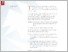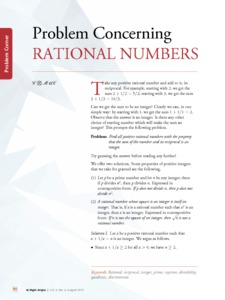# Problem concerning rational numbers

Community Mathematics Centre, CoMaC (2017) Problem concerning rational numbers. At Right Angles, 6 (2). pp. 98-102.Preview
Text - Published Version

## Abstract

ake any positive rational number and add to it, its reciprocal. For example, starting with 2, we get the sum 2 + 1 / 2 = 5 / 2; starting with 3, we get the sum 3 + 1 / 3 = 10 / 3. Can we get the sum to be an integer? Clearly we can, in one simple way: by starting with 1, we get the sum 1 + 1 / 1 = 2. Observe that the answer is an integer. Is there any other of starting number which will make the sum an standard choice geometry syllabus at the integer? This prompts the following problem. uler and compass is part of the e is a standard procedure for doing the job, and it is so simple Problem: Find all positive rational numbers with the property sum of the if number ut to think of an alternative to it that is just that as the simple, not and its reciprocal is an integer. a procedure, announced in a Twitter post . Try guessing the answer before reading any further! A We offer two solutions. Some properties of positive integers that we take for granted are the following. (1) Let p be a prime number and let n be any integer; then: if p divides n 2 , then p divides n. Expressed in contrapositive form: If p does not divide n, then p does not divide n 2 . Angle (2) A bisector rational number whose square is an integer is itself an F D integer. That is, if x is a rational number such that x 2 is an integer, then x is an integer. Expressed in contrapositive √ form: If n is not the square of an integer, then n is not a rational number. I Solution I. Let x be a positive rational number such that x + 1 / x = n is an integer. We argue as follows. • Since x + 1 / x ≥ 2 for all x > 0, we have n ≥ 2.

Item Type: Articles in APF Magazines
Authors: Community Mathematics Centre, CoMaC
Document Language:
Language
English
Uncontrolled Keywords: Rational, reciprocal, integer, prime, coprime, divisibility, G quadratic, discriminant
Subjects: Natural Sciences
Natural Sciences > Mathematics
Divisions: Azim Premji University > University Publications > At Right Angles
Full Text Status: Public
URI: http://publications.azimpremjifoundation.org/id/eprint/1354
Publisher URL: http://apfstatic.s3.ap-south-1.amazonaws.com/s3fs-...View Item